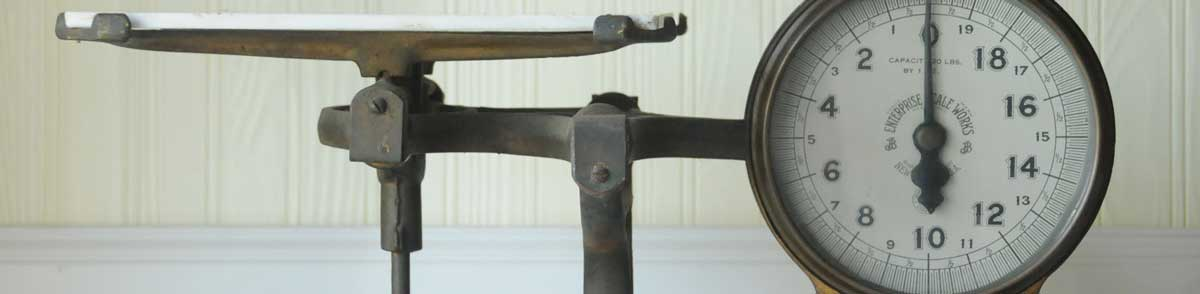# Metric Tons (Tonnes) to Carats Conversion

Enter the weight in metric tons below to get the value converted to carats.

Results in Carats:1 t = 5,000,000 ct

## How to Convert Metric Tons to CaratsTo convert a metric ton measurement to a carat measurement, multiply the weight by the conversion ratio. One metric ton is equal to 5,000,000 carats, so use this simple formula to convert:

carats = metric tons × 5,000,000

The weight in carats is equal to the metric tons multiplied by 5,000,000.

For example, here's how to convert 5 metric tons to carats using the formula above.
5 t = (5 × 5,000,000) = 25,000,000 ct

## Metric Tons (Tonnes)

One tonne is equal to 1,000 kilograms or 2,204.6 pounds, and is used throughout the world as a unit of mass/weight. One tonne is also equal to 1 megagram. The metric ton should not be confused with short tons, used primarily in the US, or long tons, used primarily in the UK.

The metric ton is an SI accepted unit for weight for use with the metric system. A metric ton is sometimes also referred to as a tonne. Metric tons can be abbreviated as t, and are also sometimes abbreviated as T, Te, or MT. For example, 1 metric ton can be written as 1 t, 1 T, 1 Te, or 1 MT.

In the US the unit is referred to as the metric ton, while the rest of the world refers to it as the tonne. Most often it's still pronounced as tun, but it is sometimes pronounced tunny.

## Carats

The carat is a unit of mass/weight equal to 200 milligrams.

A carat is sometimes also referred to as a metric carat. Carats can be abbreviated as ct, and are also sometimes abbreviated as CD. For example, 1 carat can be written as 1 ct or 1 CD.

## Metric Ton to Carat Conversion Table

Metric ton measurements converted to carats
Metric Tons Carats
0.000001 t 5 ct
0.000002 t 10 ct
0.000003 t 15 ct
0.000004 t 20 ct
0.000005 t 25 ct
0.000006 t 30 ct
0.000007 t 35 ct
0.000008 t 40 ct
0.000009 t 45 ct
0.0000001 t 0.5 ct
0.000001 t 5 ct
0.00001 t 50 ct
0.0001 t 500 ct
0.001 t 5,000 ct
0.01 t 50,000 ct
0.1 t 500,000 ct
1 t 5,000,000 ct